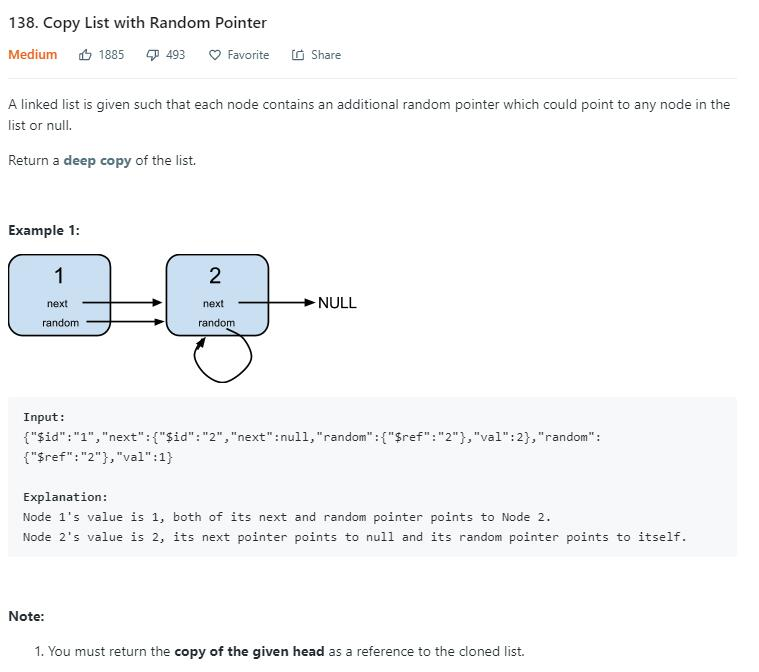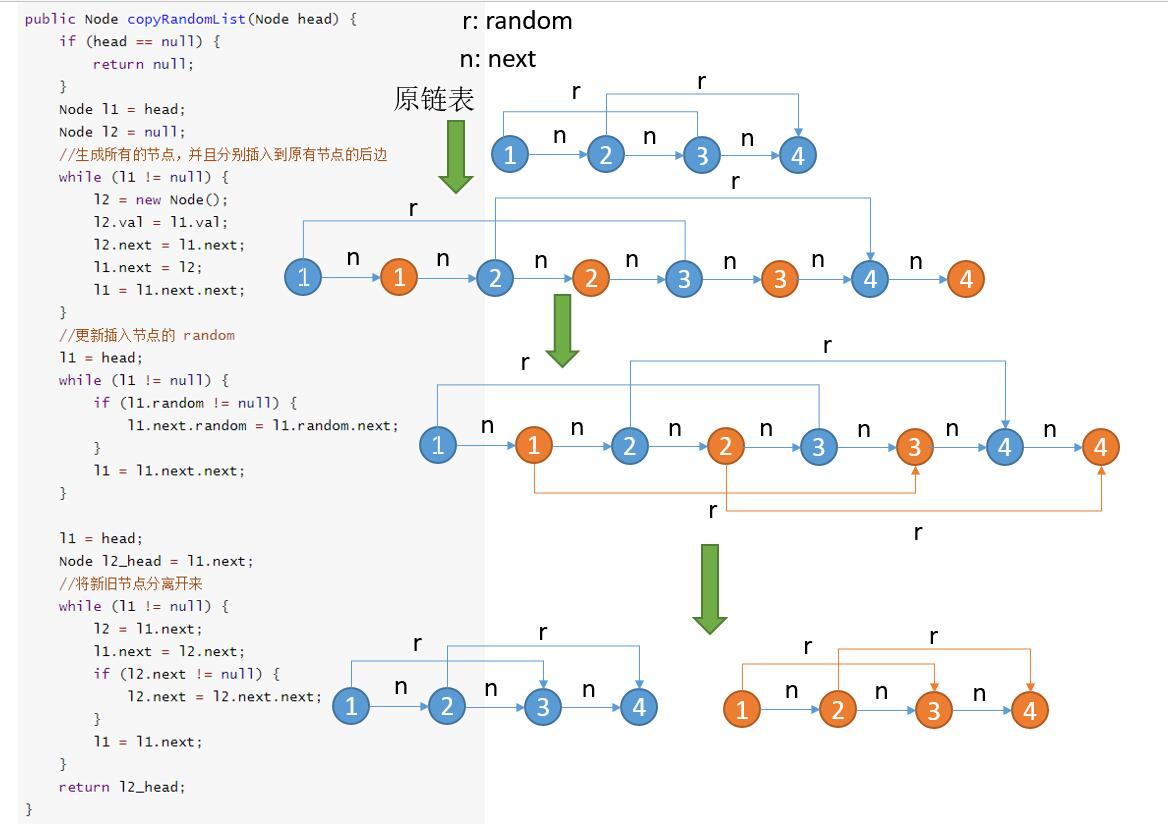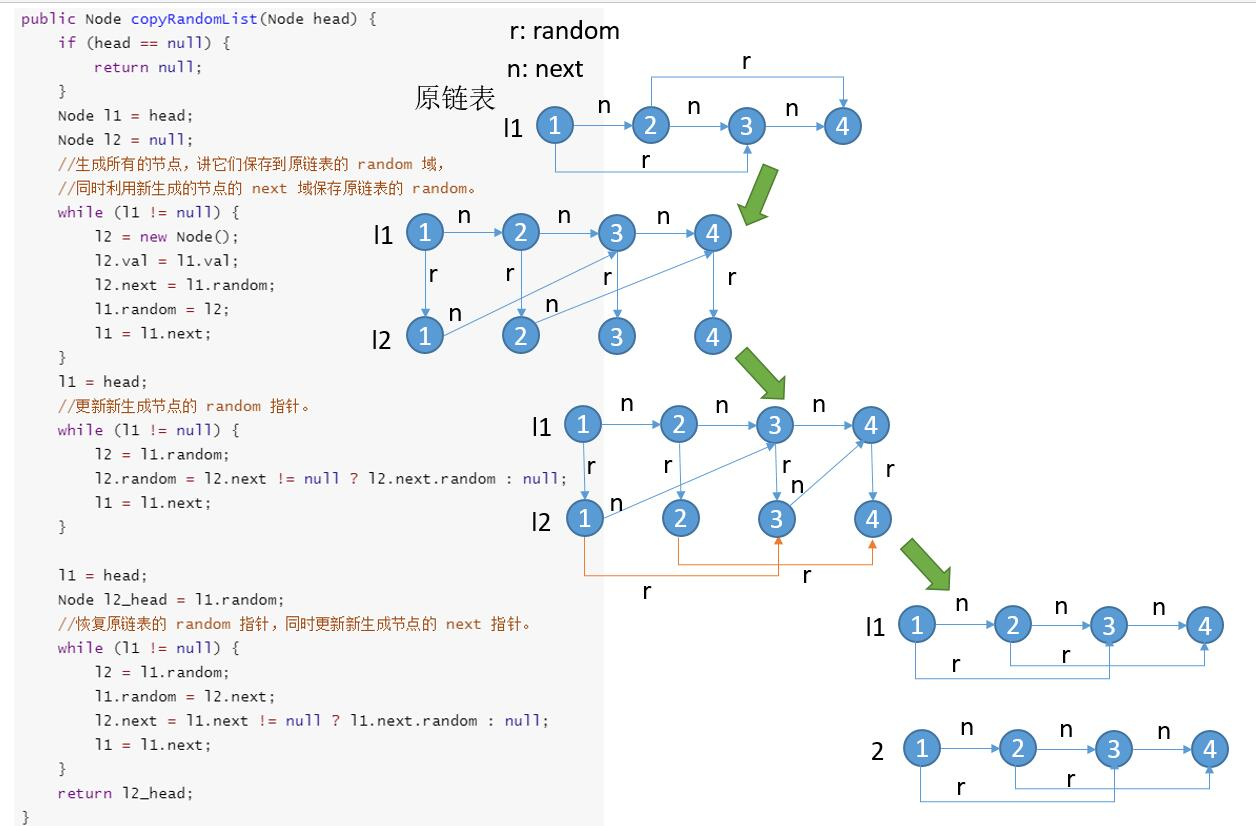# 题目描述（中等难度）# 思路分析

133 题 一样，我们可以利用 HashMap 将节点提前生成并且保存起来，第二次遍历到他的时候直接从 HashMap 里边拿即可。

2020.3.3 更新，leetcode 增加了样例，之前没有重复的数字所以 key 存的 val ，现在有了重复数字，将 key 修改为 Node。此外 Node 的无参的构造函数也被去掉了，也需要修改。

# 解法一

public Node copyRandomList(Node head) {
return null;
}
HashMap<Node, Node> map = new HashMap<>();
while (h != null) {
Node t = new Node(h.val);
map.put(h, t);
h = h.next;
}
while (h != null) {
if (h.next != null) {
map.get(h).next = map.get(h.next);
}
if (h.random != null) {
map.get(h).random = map.get(h.random);
}
h = h.next;
}
}


# 解法二

1 -> 2 -> 3

1 -> 2
^
c

1 -> 2 -> 3
^
c



public Node copyRandomList(Node head) {
return null;
}
HashMap<Node, Node> map = new HashMap<>();
Node cur = new Node(-1); //空结点，dummy 节点，为了方便头结点计算
while (h != null) {
//判断当前节点是否已经产生过
if (!map.containsKey(h)) {
Node t = new Node(h.val);
map.put(h, t);
}
//得到当前节点去更新它的 random 指针
Node next = map.get(h);
if (h.random != null) {
//判断当前节点是否已经产生过
if (!map.containsKey(h.random)) {
next.random = new Node(h.random.val);
map.put(h.random, next.random);
} else {
next.random = map.get(h.random);
}

}
//将当前生成的节点接到 cur 的后边
cur.next = next;
cur = cur.next;
h = h.next;
}
}


# 解法三

1. 生成所有的节点，并且分别插入到原有节点的后边
2. 更新插入节点的 random
3. 将新旧节点分离开来public Node copyRandomList(Node head) {
return null;
}
Node l2 = null;
//生成所有的节点，并且分别插入到原有节点的后边
while (l1 != null) {
l2 = new Node(l1.val);
l2.next = l1.next;
l1.next = l2;
l1 = l1.next.next;
}
//更新插入节点的 random
while (l1 != null) {
if (l1.random != null) {
l1.next.random = l1.random.next;
}
l1 = l1.next.next;
}

//将新旧节点分离开来
while (l1 != null) {
l2 = l1.next;
l1.next = l2.next;
if (l2.next != null) {
l2.next = l2.next.next;
}
l1 = l1.next;
}
}


# 解法四

1. 生成所有的节点，将它们保存到原链表的 random 域，同时利用新生成的节点的 next 域保存原链表的 random
2. 更新新生成节点的 random 指针。
3. 恢复原链表的 random 指针，同时更新新生成节点的 next 指针。public Node copyRandomList(Node head) {
return null;
}
Node l2 = null;
//生成所有的节点，讲它们保存到原链表的 random 域，
//同时利用新生成的节点的 next 域保存原链表的 random。
while (l1 != null) {
l2 = new Node(l1.val);
l2.next = l1.random;
l1.random = l2;
l1 = l1.next;
}
//更新新生成节点的 random 指针。
while (l1 != null) {
l2 = l1.random;
l2.random = l2.next != null ? l2.next.random : null;
l1 = l1.next;
}

//恢复原链表的 random 指针，同时更新新生成节点的 next 指针。
while (l1 != null) {
l2 = l1.random;
l1.random = l2.next;
l2.next = l1.next != null ? l1.next.random : null;
l1 = l1.next;
}